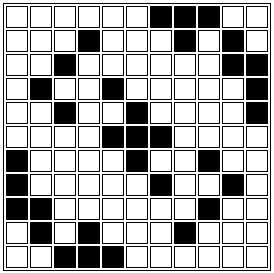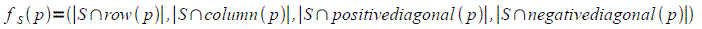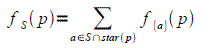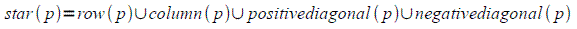27 April 2009Constant width figure of type (11,11,3)

p.. We will say a collection of squares on an nxn board is a figure of constant width w if every row, column and diagonal (both main diagonals) intersects the collection in exactly w squares or does not intersects it at all. Such a figure will contain exactly k*w squares and we will say it is of type @(n,k,w)@. Note that the figures of type @(n,n,1)@ are the solutions to the n-queens problem.

Finding figures of constant width with the computer is an interesting problem. This problem was first described here.

The following is a backtracking algorithm that enumerates all connected figures of type @(n,_,w)@.

I use the word connected in the following sense. Imagine each point in the figure is a teleportation unit and you are allowed to jump between any two points laying on the same row or column or any of the two main diagonals. A figure will be connected if there is a path of jumps between any two points in it.

For example, the n-queens solutions (figures of constant width 1) are totally disconnected; so this program does not find them.

We will start by defining, for every finite subset S of the infinite board, the functionsuch that:where p is point on the board, row(p) is the row containing p, column(p) the column containing p and so on.

This function is locally defined, meaning that it satisfies the following expression:where :The algorithm assumes fS(p)=(w,w,w,w) for every p belonging to the solution S, it then attempts to recursively satisfy the above mentioned locally defined property. This process either converges to a figure of type (n,_,w) or escapes the nxn board boundary.

Here is the link to the full F# program (cwfigures.fs) and bellow is the function @find@ that performs the search strategy.

``````let find len F availables buffer =
let rec search sol len (avlen,avail) (bufflen,buff) =
match buff with
|_ when avlen + bufflen < len -> () //not enough left to complete a solution
|[]      -> F sol //found one!
|p::tail -> let (r,rows), (c,columns), (d1,diag1), (d2,diag2), remaining = collect p avail
if     r  < state.r  p
|| c  < state.c  p
|| d1 < state.pd p
|| d2 < state.nd p then () //can not satisfy local constraint
else
(search (p::sol) (len-1)
|> (prune_before_continue remaining
>> combinator diag2   (state.nd p)
>> combinator diag1   (state.pd p)
>> combinator columns (state.c  p)
>> combinator rows    (state.r  p)
)) (bufflen - 1, tail)
search [] len availables buffer;;

``````

While there are other backtracking strategies, some of them more aesthetically appealing, this was the faster combination I could come up with. To generate all figures of type (11,_, 3) it took roughly 31 days on a Windows XP virtual machine hosted on Linux running on a quad-core. The same program running on the Linux host (with Mono 2.0.1) performed about twice slower.

There are 21 solutions of type (11,11,3) and 1 solution of type (11,10,3), module the symmetries of the square. You can see them here